# 7 Best electrical calculator apps for android and their features

What is electrical calculators

Electrical calculators are widely used to get a safe electrical design, electrical accidents can be prevented by the help of electrical calculators. In order to prevent electrical accidents, we must know certain details like the capacity of the main circuit breaker and the size of the wire. By using the electrical calculators we could do the household wiring, electrical engineers depends a lot on electrical calculators for the electrical installations and design. If there is any mistake in the electrical wiring it could do a lot of damage to the equipment and it could also pay the way for fire hazards so electrical calculators do a great role in designing electrical circuits.

1.Electrical calculations app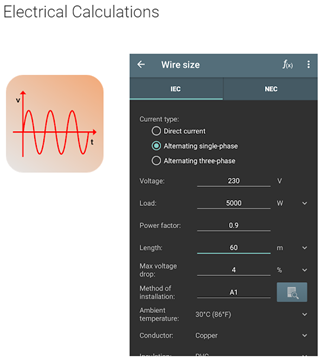The electrical calculation is a great calculator for the electrical section it is an app that can be downloaded from the google-play, it can be downloaded by using our smartphones and this app has multiple language features. It has about 30 language features. By this app we calculate the size of the wire, voltage drop and calculation of active, reactive, and apparent power can be done by this app and there is a lot of other features too.

What are the special features of electrical calculations app

• Calculation of voltage drop
• Calculation of current
• It can calculate the apparent, active and reactive power
• Voltage and resistance can be calculated
• It could calculate the current carrying capacity of busbar, insulated and bare conductors
• It can be used to determine the resistor and inductor color code and the power losses in cables and lot more features

2.Electrical calculator app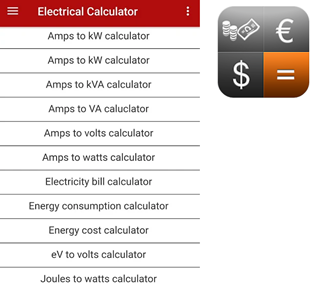This electrical calculator app can be very useful for students and electrical engineers too. It can be used to calculate the most important electrical sizes, electrical power, electrical resistance, and electrical charge and current. We don’t need to remember all the complex formulas while using this app and this app can be downloaded from Google play store. This app has all the electrical engineering calculations and conversions which include voltage, current, and power efficiency. This calculator app has much electrical power formula and many conversion features, we only need to enter the value and we could get the result

What are the special features of electrical calculator app

• Electricity bill can be calculated
• Energy cost calculator
• Voltage divider and voltage drop calculator
• It has electrical formulas and electrical load calculator
• Delta/star impedance conversion
• It can do the watts to horsepower conversion
• Power factor, lighting and transformer calculations and lot more features

3. Electrical calculator and formula app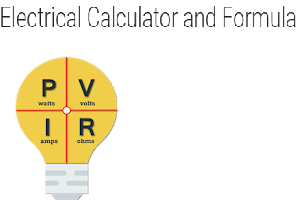This electrical calculator app will be useful to calculate electrical engineering formulas in a simple way and this app has formulas with the description too, this app can be downloaded from Google play store. Its important feature is that it can be used to calculate the generator sizing for different motor loads. Wire size can be calculated in this app it can calculate the wire size of a single and three-phase connection and the values of a resistor, capacitor and inductors can be calculated in series and parallel. Three-phase power, voltage, and current can be calculated and conversions like a star to the delta, delta to star, horsepower to KW conversion and lot more can be done.

What are the special features of Electrical calculator and formula app

• Wire size and SMD register code calculation
• Values of power, resistance and current can be calculated, and the calculation of voltage can be done by power and resistance, power and current, current and resistance
• Resistor, capacitor, and inductors values can be calculated in series and parallel
• Single-phase and three-phase calculations
• Color code of inductors and resistors can be done
• Conversions like delta to star and star to delta and lot more can be done by this app

4. Volts watts and amps calculator app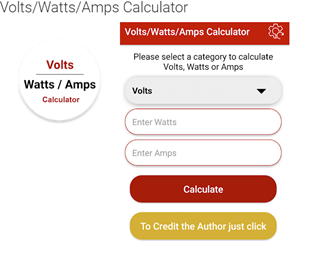By using this electrical calculator app we can calculate the voltage, watt, and ampere. Watt is the unit of power by calculating the watts we could know how much power an electric device uses per second. Ampere is the measurement of electric current and it represents the electricity flow rate and volt is the measurement of voltage and it represents the electrical potential difference or pressure.

5. Electrical calculator

This electrical calculator app can be used to do electrical calculations and conversions which includes voltage, current and power efficiency, resistor-capacitor and inductor combinations, resonant frequency, reactance, 4-band, 5-band, and 6-band resistor color coding, inductor color coding, delta/star impedance conversion, power factor, and transformer calculations

What are the special features of Electrical calculator app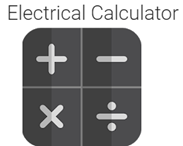• Joules to watts calculator
• Ohm’s law calculator
• Voltage divider and drop calculator
• Wire gauge calculator

6. All electrical formulaThis app is very useful for students and engineers they can learn how to use an electrical formula, this application is also suitable for people who like to do electronic and electrical calculations. This app has many formulas like single-phase to a three-phase converter, electrical and power formulas. This app contains all the electrical engineering calculations and conversions which include voltage, current, power, and efficiency. The major use of an electrical app is that we would be able to calculate the most important electrical sizes.

What are the special features of all electrical formula

• It can be used to calculate electrical power, electrical resistance, and electrical charge.
• It has all electrical formulas, power formulas, power equation, and current formula
• It has basic electrical formulas, unit of electrical power, electricity unit calculator and electric converter
• They can be used to convert amps to KW and ac to dc power conversion in single three-phase and lot more.

7. Electrical calculations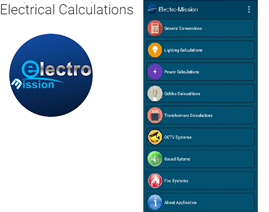This app can be used to do the conversions like lighting calculations, power calculations, and they can be used to calculate the power cable which will be really helpful for the electrical design. It can do the frequency-related conversions

What are the special features of electrical calculations

• It can be used for CCTV surveillance, CCTV lenses calculator and can be used for DVR
• It can be used for transformer calculations
• It can be used for cable size calculations, cable depth and distance correction factor
• It can also be used in power calculations, lighting calculations like watt, lumen, candela, lux…